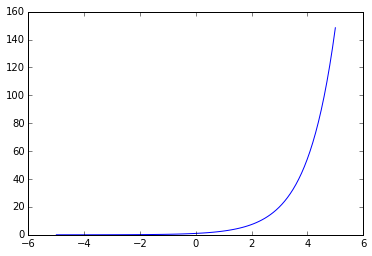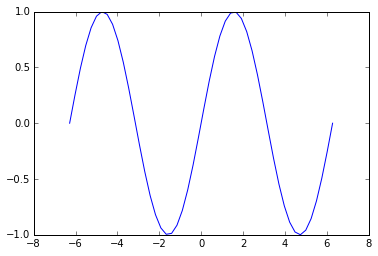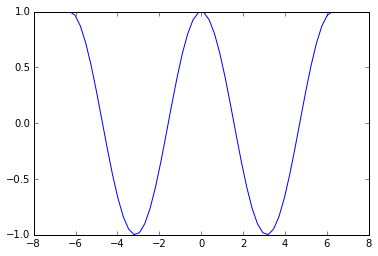# 函数

## 一些函数的例子：

1.多项式(polynomials)def f(x):
return x**3 - 5*x**2 + 9


    print f(3)
-9
print f(1)
5


    import numpy as np
x = np.linspace(-5, 5, num = 100)
y = f(x)
import matplotlib.pyplot as plt
plt.plot(x,y)2.指数函数(Exponential Functions):def exp(x):
return np.e**x

print exp(2)
7.3890560989306495


    print np.exp(2)
7.3890560989306495


    plt.plot(x, exp(x))def exp2(x):
sum = 0
for k in range(100):
sum += float(x**k)/np.math.factorial(k)
return sum

print exp(1), exp(2), exp(3)
2.718281828459045 7.38905609893 20.0855369232

print exp2(1), exp2(2), exp2(3)
2.7182818284590455 7.38905609893 20.08553692323.对数函数(Logarithmic Functions):numpy为我们提供了以为底的对数函数：

    x = np.linspace(0,10,100,endpoint = False)
y1 = np.log2(x)
y2 = np.log(x)
y3 = np.log10(x)
plt.plot(x,y1,'red',x,y2,'yellow',x,y3,'blue')4.三角函数(Trigonometric Functions):

    plt.plot(np.linspace(-2*np.pi,2*np.pi),np.sin(np.linspace(-2*np.pi,2*np.pi)))plt.plot(np.linspace(-2*np.pi,2*np.pi),np.cos(np.linspace(-2*np.pi,2*np.pi)))